# OECD Tourism flows data analysis 6 - Testing for serial correlation of the error term using RUnsplashBob Brewerが撮影した写真

This post is following of the above post.

By previous posts, I made 6 models. These models are time series regression model.

So, I would like to check whether there are serial correlation of the error term.

First, I load dynlm package and lmtest package.

Next, I use resid() function to get residuals(= error terms), ts() function to make residulas be time series object, dynlm() fundtion for regression analysis and coeftest() function to test regression results.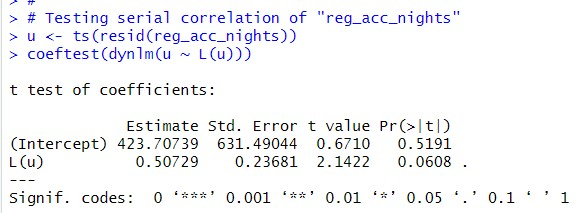For reg_acc_nights model, p-value of L(u) is 0.0608, so there is not serail correlation.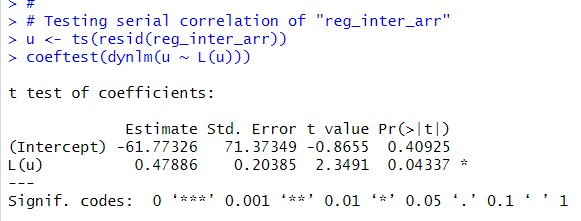For reg_inter_arr model, L(u) p-value is 0.04337, so I can say there is serial correlation.

Before checking for reg_inter_dep, I will make custom function to test serial correlation.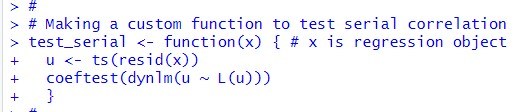Okay, let's use test_serial() function for reg_inter_dep.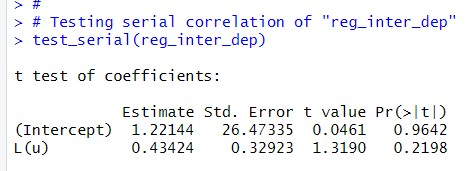p-value is 0.2198, so I can say there is not serial correlation for reg_inter_dep.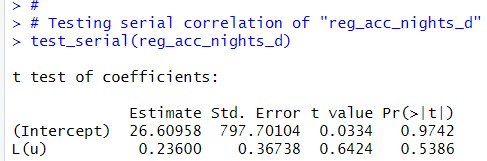p-value is 0.5386, so I can say there is not serial correlation for reg_acc_nights_d.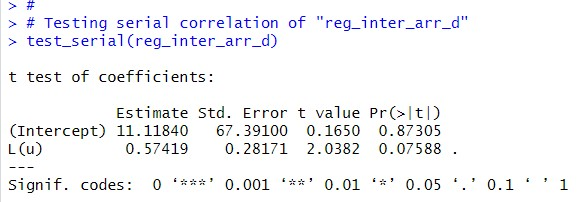p-value is 0.07588, which is greater than 0.05. I can say there is not serial correlation.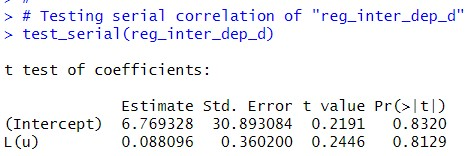p-value is 0.8129, so I can say there is not serial correlation.

So, "reg_inter_arr" model has serial correlation with p-value: 0.4337 and "reg_inter_arr_d" model seems have serial correlation with p-value: 0.07588 and "reg_acc_nights" model seems have serial correlation with p-value: 0.0608.

That's it. Thank you!

Next post is

To read from the 1st post,